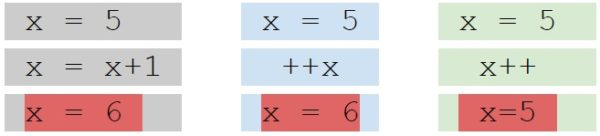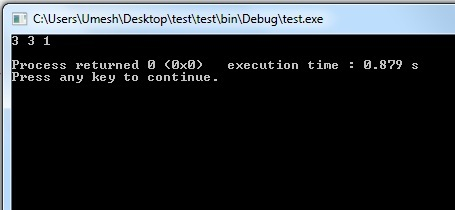Monday , May 20 2019

# Operators in C Programming

C Programming Language support several operators to perform different operations. These operators in c programming are mostly in the form of symbols which used to perform logical and arithmetic operations. These operators generally work on many types of variables and constants although some are restricted to work on specific types. In between these operators most operators are binary, meaning they take two operands and few are unary (only take one operand). Operators can be classified based on type of operations they perform.

#### Arithmetic Operators

Arithmetic C operators are used to perform mathematical operations such as addition, subtraction, multiplication and division on numerical values (variables and constants).

 Operators Operations Example + Addition a+b – Subtraction a-b * Multiplication a*b / Division a/b % Modulus a%b

EXAMPLE 1: Demonstrate working on Arithmetic Operators. In this example two values “6” and “4” used to perform arithmetic operations such as addition, subtraction, multiplication, division, modulus and output displayed for each output.

#### Increment & Decrement Operators

C Programming has two very useful operators increment (++) and decrement (–). Increment operators are used to increase the value of variable by one and decrement operators are used to decrease the value of the variable by one in C Programs.

Increment Operators: ++i, i++
Decrement Operators: –i, i –

 Operator type Operations Description Pre increment ++i Value of i is incremented before assigning it to variable i. Post increment i++ Value of i is incremented after assigning it to variable i. Pre decrement –i Value of i is decremented before assigning it to variable i. Post decrement i– Value of i is decremented after assigning it to variable i.

Before going further to discuss increment and decrement operators, let understand some expressions; that are being used in all operators. Now some issues of pre and post increment and decrement, we will discuss after solve following programming:Increment and Decrement Operator in C Language

In above program you might have notice that value of x increase to one in all three expression but after the increasing one, the final value of x in not same, third box (++x) result is differ to another two boxes (x++). Now you know that c operators are, ++ and — operators are Unary operators. Post increment or decrement are lowest precedence so it is solve at the end of program. Hence above program in third box first value of x is print i.e. 5 after, it is increase. Unary operators are solve right to left.

Explanation: C’s calling convention is from right to left. That is, firstly 1 is passed through the expression i++ and then i is incremented to 2. Then result of ++i is passed. That is, i is incremented to 3 and then passed. Finally, latest value of i, i.e. 3, is passed. Thus in right to left order, 1,2,3 get passed. Once printf() collects them, it prints them in the order in which we have asked it to get them printed(and not the order in which they were passed). Hence, output will be 3 3 1 is printed.Output of Increment and Decrement Operator in C Programming

#### Assignment Operators

C has several assignment operators available– one simple assignment operator and several convenience assignment operators that combine arithmetic or bitwise operations with assignment. The operators are as follows:

 Operator Description Example = Simple assignment operator. Assigns values from right side operands to left side operand c = a + b will assign the value of a + b to c += Add AND assignment operator. It adds the right operand to the left operand and assign the result to the left operand. c += a is equivalent to c = c + a -= Subtract AND assignment operator. It subtracts the right operand from the left operand and assigns the result to the left operand. c -= a is equivalent to c = c – a *= Multiply AND assignment operator. It multiplies the right operand with the left operand and assigns the result to the left operand. c *= a is equivalent to c = c * a /= Divide AND assignment operator. It divides the left operand with the right operand and assigns the result to the left operand. c /= a is equivalent to c = c/a %= Modulus AND assignment operator. It takes modulus using two operands and assigns the result to the left operand. c %= a is equivalent to c = c % a <<= Left shift AND assignment operator. c <<= 2 is same as c = c << 2 >>= Right shift AND assignment operator. c >>= 2 is same as c = c >> 2 &= Bitwise AND assignment operator. c &= 2 is same as c = c & 2 ^= Bitwise exclusive OR and assignment operator. c ^= 2 is same as c = c ^ 2 |= Bitwise inclusive OR and assignment operator. c |= 2 is same as c = c | 2

Example Program for Assignment Operator in C:

Explanation: The value 8.3 constant is converted to an int and then stored in a. Here at line a %= 4;  a is assigned to the remainder left over when a is divided by 4. Also this line shows multiple assignments on a single line. The c = 5 is done first, then the value of c (which is now 5) is assigned to a.

Bitwise operations will be covered later but here is a brief explanation. At line a <<= c 3; the c – 3 operation gives 2, so the statement becomes a <<= 2. The a variable at that point is 5 (101 in binary), which when left shifted by 2 becomes 20 (10100 in binary). The bitwise and operation at line a &= c; keeps only the 1 bits that are common to both numbers. So a (20 decimal, 10100 binary) bitwise and with c ( 5 decimal, 101 binary ) results in 4 ( 100 binary ), which is then stored in a.

#### Relational Operators

Relational operators are used to find the relation between two variables. i.e. to compare the values of two variables in a C program.

 Operator Description Example > a is greater than b a > b < a is less than b a < b >= a is greater than or equal to b a >= b <= a is less than or equal to b a <= b == a is equal to b a == b != a is not equal to b a != b

Example Program for Relational Operator

#### Logical Operators

These operators are used to perform logical operations on the given expressions. When we use if, for, while then use one condition at once time what happen if we examine condition more than one? In this case we use logical operators. So logical operators are used to combine two or more condition. There are 3 logical operators in C language. They are, logical AND (&&), logical OR (||) and logical NOT (!).

 Operator Description Example && Logical AND. True only if all operands are true If a = 1 and b = 0 then (a && b) is False || Logical OR. True only if either one operand is true If a = 1 and b = 0 then (a || b) is True ! Logical NOT. True only if the operand is 0 If a = 1 then !(a) is False

Some numerical example:

Now let’s have some discussion on NOT operator when we write condition if( 1 ! = 0 ) what is mean it? It is mean “one is not equal to zero“, so is condition TRUE or False? It is true.

Let’s understand following example:

In above example evaluate first printf  if condition is false otherwise evaluate second printf.

Example of Logical Operator

Explanation of logical operator program:

• (a = b) && (c > 5) evaluates to 1 because both operands (a = b) and (c > b) is 1 (True).
• (a = b) && (c < b) evaluates to 0 because operand (c < b) is 0 (False).
• (a = b) || (c < b) evaluates to 1 because (a = b) is 1 (True).
• (a != b) || (c < b) evaluates to 0 because both operand (a != b) and (c < b) are 0 (False).
• !(a != b) evaluates to 1 because operand (a != b) is 0 (False). Hence, !(a != b) is 1 (True).
• !(a == b) evaluates to 0 because (a == b) is 1 (True). Hence, !(a == b) is 0 (False).

#### Conditional Operators

Conditional operators return one value if condition is true and returns another value is condition is false. This operator is unusual in that it takes three operands. The syntax of this operator is like this: Condition? True_Value: False_Value;

Example: (A>100? 0:1);

In this example, if A is greater than 100, 0 is returned else 1 is returned. This is equal to if else conditional statements.

Example of Conditional Operator:

#### Bitwise Operators

Bitwise operators perform manipulations of data at bit level. These operators also perform shifting of bits from right to left. Bitwise operators are not applied to float or double.

 Operator Description & Bitwise AND | Bitwise OR ^ Bitwise exclusive OR << Left Shift >> Right Shift

Now let’s see truth table for bitwise &, | and ^:

 A B A & B A | B A^B 0 0 0 0 0 0 1 0 1 1 1 0 0 1 1 1 1 1 1 0

The bitwise shift operators shift the bit value. The left operand specifies the value to be shifted and the right operand specifies the number of positions that the bits in the value are to be shifted. Both operands have the same precedence.

EXAMPLE: Bitwise Operators in C

a = 0001000
b= 2
a << b = 0100000
a >> b = 0000010

#### Special Operators

Sizeof() operator calculate the size of data i.e. how many bit a specific data having.

Syntax of sizeof operator: printf(“<format string>”,sizeof<(data type variable)>);

Example of Sizeof Operator:

The output from this program prints “4” because my machine is 64-bit and size of integer variable (i.e. ‘x’) on 64-bit machine is 4-bytes. We already have covered in variable and data type in C article.

That’s all for this post and now we are familiar with most of Operators in C Programming Language. In next post we will learn about decision making statements i.e. If Statements in C Programming. Feel free to drop comment. Thanks !

### About Umesh LokhandeUmesh Lokhande holds a Master degree in Scientific Instrumentation from University of Applied Sciences Jena, Germany. and has previously worked at Orbotech, Alere Technologies etc. Umesh is also a founder and first author of BINARYUPDATES.COM